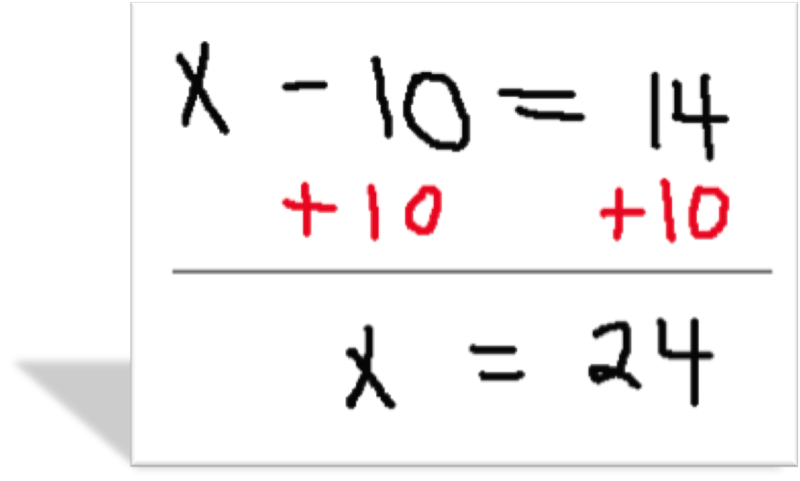# Solving Equations by Adding or SubtractingWe use what mathematicians call inverse operations. An inverse operation is an operation that undoes another operation. Addition and subtraction are inverse operations for each other. For example, the inverse of adding 5 is subtracting 5. The inverse of subtracting 7 is adding 7.

Take a moment and think about it and relate it to prior knowledge.

7 + 6 = 13

13 – 7 = 6

These are related facts!

Now we know that inverse operations are what we use to help us solve equations. How can we solve equations using inverse operations?

To solve equations, you have to perform inverse operations to get the variable by itself. Remember – addition and subtraction are inverse operations.   If an equation contains addition, solve it by subtracting from both sides to undo the addition.   Similarly, if an equation contains subtraction, solve it by adding to both sides to undo the subtraction.

For example. If we had x + 5, then we could subtract 5 and get x by itself. A number plus its inverse is just 0.

10 – 10 = 0  and -7 + 7 = 0

Let’s take a look at the definition of addition, subtraction, multiplication, and division equations.

To solve an addition equation, one must subtract the same number from each side of the equation so that the variable will be by itself on one side of the equation and the answer on the other side.

The two sides are equal. Think of it like a balance. If you take something away from one side, the two sides would no longer be equal, no longer in balance. But, if you take the same amount away from both sides, they stay equal.

Example:This equation was 2 + 5 = 7. We took 5 away from each side and got 2 = 2, which, of course, is true!

## Solving a Subtraction Equation

To solve a subtraction equation, one must add the same number from each side of the equation so that the variable will be by itself on one side of the equation and the answer on the other side.

This works because -10 + 10 = 0. Adding the inverse makes the number go away!

Hopefully, you noticed in the definitions that inverse operations were used to solve.

Example:(source)